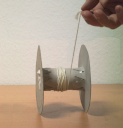#Theoretical Mechanics IPSP

Jürgen Vollmer, Universität Leipzig

### Site Tools

book:chap4:eom-ode

# 4. Motion of Point Particles

In Chapter 3 we learned how to set up a physical model based on finding the forces acting on a body, and thus determining the acceleration of its motion. For a particle of mass~$m$ and position~$\vec q$ Newton's second law relates its acceleration $\ddot{\vec q}$ to the force that is acting on the particle. In Chapter 2 we saw that the total force $\vec F( \vec q, \dot{\vec q}, t )$ acting on the particle may depend on $\vec q$, $\dot{\vec q}$, and $t$. The resulting relation between the acceleration and the force is called equation of motion of the particle, Definition 3.3. In the present chapter we will discuss approaches that will allow us to systematically find the solutions of EOMs. Moreover, we will explore what type of behavior is encountered for different types of initial conditions.

At the end of this chapter we will discuss the motion of planets around the sun, moons around their planets, and will be able to figure out which rules determine the intricate trajectory of 'Oumuamua shown in Figure 3.12.

The PDF file of the chapter is available here.